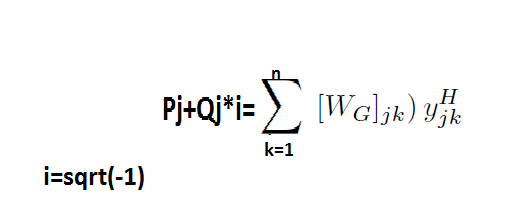# Complex equality constraints

Hello to all! My question is if i declare a variable as complex then cvx takes as variables the angle and the absolute value by default?An illustrative example is above.

variable w comple

subject to
(1+5i)*w==4+3i

The cvx will make 2 equations/constraints for the angle and the absolute value or i must declare angle and asb value as two different variables?

One or both sides of an equality constraint may be complex; inequality constraints, on the other hand, must be real. A complex equality constraint is equivalent to two real equality constraints, one for the real part and one for the imaginary part. An equality constraint with a real side and a complex side has the effect of constraining the imaginary part of the complex side to be zero.So have i defined well the above equation with this code?

P(j)==real(conj(ybus(j,j))*W(j,j)+dot(W(j,j+1:n),conj(y(j,j+1:n))));
Qg(j)==imag(conj(ybus(j,j))*W(j,j)+dot(W(j,j+1:n),conj(ybus(j,j+1:n))));

You can write a single equality constraint without explicitly extracting the real and imaginary parts of either side of the equality constraint. Of course, each side of the equality constraint must be affine.

hmm ok but i need P and Q as two separately variables…so is it correct right? another question is if i could use doubly inequality for example a<=x<=b!!

P and Q can be separate variables.

You can use double inequality. However, as mentioned in the users’ guide extract above, both sides of each inequality constraint must be real.

thank you very much for your support!!i forgot to mention that W matrix is a complex variable not a known matrix!!!

Just make sure you follow all of CVX"s DCP rules. If y is not a CVX variable, you should be o.k.

thank you!! y is a constant so maybe the mistake is in theory and not at the syntax…

another one question. i saw in the forum and manual that i can use for loop to set my constraints, but matlab gives me the following error;/
Error: File: mpopfcase6.m Line: 21 Column: 14
Illegal use of reserved keyword “for”.

my code is the above:
function [W,Pg]=mpopfcase6(cir,n);
%define all the constants, limits etc
ybus=full(makeYbus(cir));
g=graph(abs(ybus));
Pload=[0 0 0.7 0 0 0.7];
Qload=[0 0 0.7 0 0 0.7];
cvx_begin
variable W(n,n) complex semidefinite;
variable Pg(n);
variable Qg(n);
minimize 0.1*(Pg(1))+0.3*Pg(3) ;
subject to
[

``````          for i=1:n
end

for i=1:n
end

W==semidefinite(n);
W(1,1)==1;
Pg(2)==0;  Pg(4)==0; Pg(5)==0; Pg(6)==0;
Qg(2)==0;  Qg(4)==0; Qg(5)==0; Qg(6)==0
]
cvx_end

end``````

It looks like you have an extraneous
`[`
in the line after
`subject to`
and before
`for i=1:n`

Also, remove the
`]`
before
`cvx_end`

You don’t have to group your constraints, and you really don’t need
`subject to`
which is basically ignored by CVX and MATLAB, and only serves as an aid to human understanding of the code.